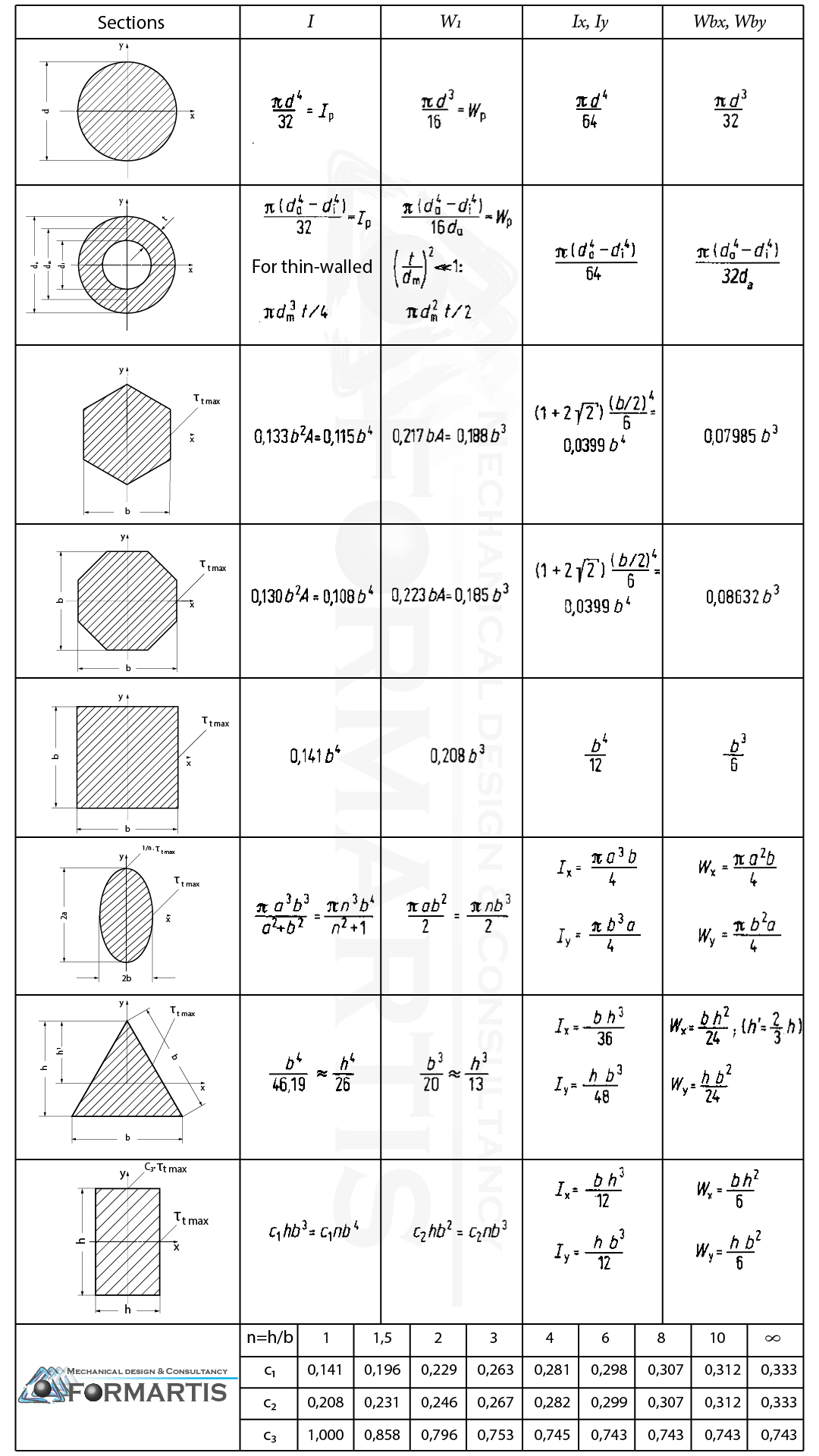## #FORMARTISTIPS n.3

### MOMENTS OF INERTIA OF A SECTIONWHEN WE'RE IMPRESSING FORCE TO A BODY WITH MASS, ONCE THE FRICTIONS HAVE BEEN OVERCOME, IT WILL BE SUBJECTED TO A CERTAIN ACCELERATION. THIS ACCELERATION WILL BE INVERSELY PROPORTIONAL TO ITS MASS.

In Physics, mass is the measurement that determines the inertia of a body relative to a shift, that is, its ability to oppose a variation in its state of stillness or motion.

The greater the mass of a body, the less acceleration we will be able to give.

As this goes for translational motions, so in rotary motions there’s a measurement similar to mass, the moment of inertia. The moment of inertia is defined as the product of mass and the square of the distance between reference axis and the centroid of the section. It represents the ability of the point to oppose to a rotational motion: the greater the moment of inertia, the more difficult will be to rotate the body around the chosen axis.

A body, compared to different rotation axis, will generate different moments of inertia.

In the table below some examples, assuming that each of the bodies is homogeneous.